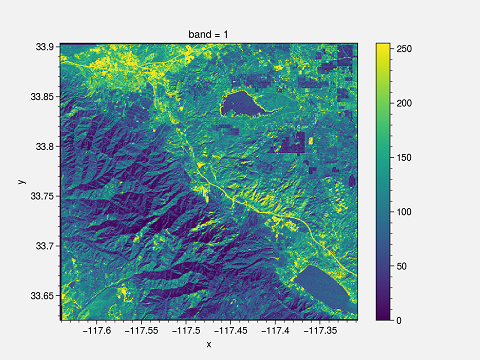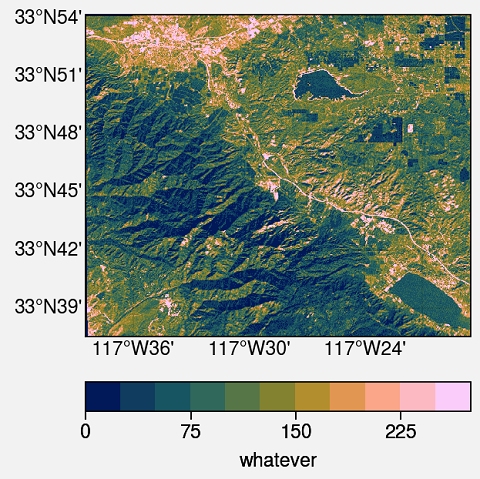# Plotting GeoTIFF in Python

### Plotting GeoTIFF in Python

Pratiman, 30 June 2020

Usually, working with geographic data, you have to use GIS software. For plotting multiple plots with the same quality might be cumbersome. Hence, some people choose to automate the process; I was also struggling to do the same. So here is a simple example of plotting a GeoTIFF file.

First of all load your dataset using `xarray.open_rasterio`.

``````import xarray as xr
dem = dem #getting the first band
``````

The easiest way to plot is by using the plot function of xarray `dem.plot()`.This plot has certain drawbacks. First of all, x and y labels are too small; the title is “band 1”, and the colorbar need to be changed. You can change it to make it suitable for publishing.

I am using ProPlot. This is an impressive library for publication-quality plots. This is the whole script and output.

## Python Script

``````import proplot as plot
import xarray as xr

dem = dem

# Define extents
lat_min = dem.y.min()
lat_max = dem.y.max()
lon_min = dem.x.min()
lon_max = dem.x.max()

#Starting the plotting
fig, axs = plot.subplots(proj=('cyl'))

#format the plot
axs.format(
lonlim=(lon_min, lon_max), latlim=(lat_min, lat_max),
land=False, labels=True, innerborders=False
)

#Plot
m = axs.pcolorfast(dem, cmap='batlow')
cbar = fig.colorbar(m, loc='b', label='whatever') #Adding colorbar with label

#Saving the Figure
fig.savefig(r'geotiff.png')
``````## Explanation

1. Basic import stuff for Python
``````import proplot as plot
import xarray as xr
``````
2. Open the GeoTIFF dataset using `xarray.open_rasterio` and get the first band of the image. You can select a different band if you have multi-band GeoTIFF.
``````dem = xr.open_rasterio('https://download.osgeo.org/geotiff/samples/pci_eg/latlong.tif')
dem = dem
``````
3. Get the extent of the dataset. If you have a global dataset, then there is no need.
``````lat_min = dem.y.min()
lat_max = dem.y.max()
lon_min = dem.x.min()
lon_max = dem.x.max()
``````
4. Create the figure and axes with projection system. Here, `proj=('cyl')` means the Cartopy `PlateCarree` projection
``````fig, axs = plot.subplots(proj=('cyl'))
``````
5. Format the plot. Here, `lonlim` and `latlim` are the zoom locations of the axes. If you are using a global dataset, then you can ignore it. Turn on/off the various geographic features such as `land, ocean, coast, river, lakes, innerborders, etc.`. To turn on the lat and long labels use `labels=True`.
``````axs.format(
lonlim=(lon_min, lon_max), latlim=(lat_min, lat_max),
land=False, labels=True, innerborders=False
)
``````
6. Finally, plot the dem using `pcolorfast`. It is good enough for fast processing of the GeoTIFF files. `pcolormesh` takes a longer time to process but can be used. The colormap used is `batlow`. For more colormaps you can check using `plot.show_cmaps()`. Save the figure to your deired location.
``````m = axs.pcolorfast(dem, cmap='batlow')
cbar = fig.colorbar(m, loc='b', label='whatever') #Adding colorbar with label

#Saving the Figure
fig.savefig(r'geotiff.png')
``````
If you would like to provide any suggestions to this post. Kindly email me at pratiman_patel@hotmail.com